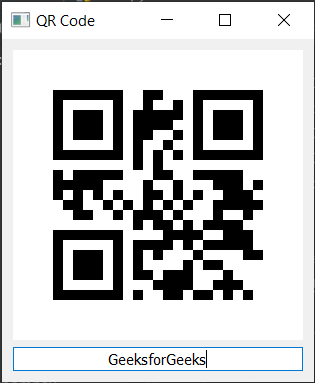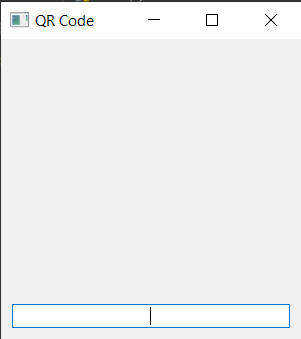# Building QR Code Generator Application using PyQt5

• Last Updated : 30 Jun, 2022

In this article we will see how we can make a QR code generator application using PyQt5. A QR code is a type of matrix barcode first designed in 1994 for the automotive industry in Japan. A barcode is a machine-readable optical label that contains information about the item to which it is attached. Below is how the application will look likeIn order to make this we will use the libraries given below
PyQt5 is cross-platform GUI toolkit, a set of python bindings for Qt v5. One can develop an interactive desktop application with so much ease because of the tools and simplicity provided by this library. Below is the command to install the PyQt5

`pip install PyQt5`

qrcode :For generating a Quick Response code is a two-dimensional pictographic code used for its fast readability and comparatively large storage capacity. The code consists of black modules arranged in a square pattern on a white background. The information encoded can be made up of any kind of data (e.g., binary, alphanumeric, or Kanji symbols). Below is the command to install the qrcode module

`pip install qrcode`

Implementation Steps :
1. Create a Image class which inherits qrcode base image
2. Inside the Image class, get the size from the border and width and override the painter event and create a initial image and fill it with white color
3. Create a main window class
4. Inside the window class create a label which will show the QR code image
5. Create a line edit to receive the text from the user
6. Add label and line edit to the vertical layout and set layout to the window
7. Add action to the line edit when entered is pressed
8. Inside the line edit action get the text of the line edit
9. Create a pixmap image of the line edit text and use Image class as image_factory
10. Set the pixmap i.e QR code image to the label

Below is the implementation

## Python3

 `# importing libraries``from` `PyQt5.QtWidgets ``import` `*``from` `PyQt5 ``import` `QtCore, QtGui``from` `PyQt5.QtGui ``import` `*``from` `PyQt5.QtCore ``import` `*``import` `qrcode``import` `sys` `# Image class for QR code``class` `Image(qrcode.image.base.BaseImage):` `    ``# constructor``    ``def` `__init__(``self``, border, width, box_size):` `        ``# assigning border``        ``self``.border ``=` `border` `        ``# assigning  width``        ``self``.width ``=` `width` `        ``# assigning box size``        ``self``.box_size ``=` `box_size` `        ``# creating size``        ``size ``=` `(width ``+` `border ``*` `2``) ``*` `box_size` `        ``# image``        ``self``._image ``=` `QImage(size, size, QImage.Format_RGB16)` `        ``# initial image as white``        ``self``._image.fill(Qt.white)`  `    ``# pixmap method``    ``def` `pixmap(``self``):` `        ``# returns image``        ``return` `QPixmap.fromImage(``self``._image)` `    ``# drawrect method for drawing rectangle``    ``def` `drawrect(``self``, row, col):` `        ``# creating painter object``        ``painter ``=` `QPainter(``self``._image)` `        ``# drawing rectangle``        ``painter.fillRect(``            ``(col ``+` `self``.border) ``*` `self``.box_size,``            ``(row ``+` `self``.border) ``*` `self``.box_size,``            ``self``.box_size, ``self``.box_size,``            ``QtCore.Qt.black)`  `# Main Window class``class` `Window(QMainWindow):` `    ``# constructor``    ``def` `__init__(``self``):``        ``QMainWindow.__init__(``self``)` `        ``# setting window title``        ``self``.setWindowTitle("QR Code")` `        ``# setting geometry``        ``self``.setGeometry(``100``, ``100``, ``300``, ``300``)` `        ``# creating a label to show the qr code``        ``self``.label ``=` `QLabel(``self``)` `        ``# creating a line edit to receive text``        ``self``.edit ``=` `QLineEdit(``self``)` `        ``# adding action when entered is pressed``        ``self``.edit.returnPressed.connect(``self``.handleTextEntered)` `        ``# setting font to the line edit``        ``self``.edit.setFont(QFont(``'Times'``, ``9``))` `        ``# setting alignment``        ``self``.edit.setAlignment(Qt.AlignCenter)` `        ``# creating a vertical layout``        ``layout ``=` `QVBoxLayout(``self``)` `        ``# adding label to the layout``        ``layout.addWidget(``self``.label)` `        ``# adding line edit to the layout``        ``layout.addWidget(``self``.edit)` `        ``# creating a QWidget object``        ``widget ``=` `QWidget()` `        ``# setting layout to the widget``        ``widget.setLayout(layout)` `        ``# setting widget as central widget to the main window``        ``self``.setCentralWidget(widget)`  `    ``# method called by the line edit``    ``def` `handleTextEntered(``self``):` `        ``# get the text``        ``text ``=` `self``.edit.text()` `        ``# creating a pix map of qr code``        ``qr_image ``=` `qrcode.make(text, image_factory ``=` `Image).pixmap()` `        ``# set image to the label``        ``self``.label.setPixmap(qr_image)`    `# create pyqt5 app``app ``=` `QApplication(sys.argv)` `# create the instance of our Window``window ``=` `Window()` `# showing window``window.show()` `# start the app``sys.exit(app.exec_())`

Output :When user entered  the text in the line edit and press enter key, QR code will be displayed and window size will get adjusted according to the size of QR codeMy Personal Notes arrow_drop_up